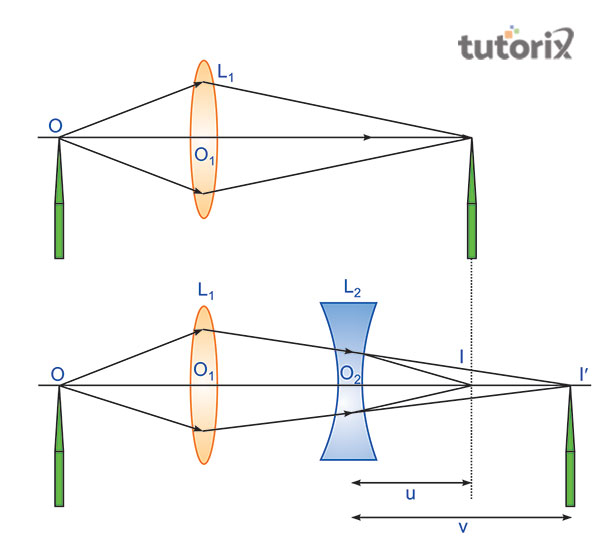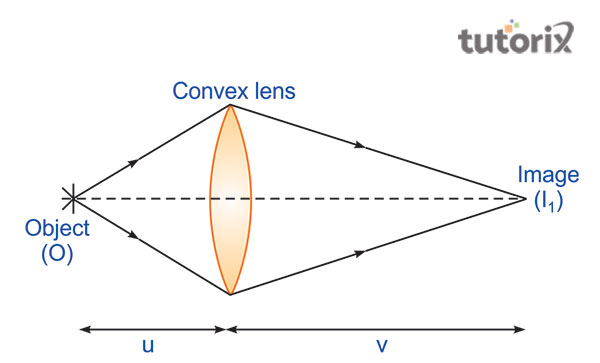# To find focal length of concave lens using convex lens

The tutorial mainly gives focuses on finding a convex mirror’s focal length and for this experiment; a convex lens is used. In this experiment, some materials are needed and they are essential for this examination. Here a bench is the most essential material and two needles are required here. It is seen that if the concave lens was not there and the object was at a distance then the focal length of the convex lens will create a real image.

## Aim

The main aim of this experiment is to find the focal length of a concave lens with the help of a convex lens.

## Required Materials

Different materials are required for this experiment such as an optical bench having four upright. A concave lens is also required for this process with a focal length (Learncbse, 2022). A convex lens and two lens holders are required for this process. A knitting needle, one thin optical needle, and one thick needle are also important materials here. Another required material is a half-meter scale.

## Theory

The theory is mainly discussed by using a formula of an experiment. It is used for the calculation of the concave lens. The formula says that f = uv/u-v.

Here f refers to the focal length of the concave lens L1. In this part, u refers to the distance of I from the optical centre of the lens L2. Lastly, v means the distance of I’ from the optical centre of the lens L2.Figure 1: Focal Length of Concave and Convex lens

## Ray Diagram and Procedure

The lab procedure goes by certain steps and for this; one should have to keep the convex lens of a known focal length in contact with the concave lens. Here the focal length of the concave lens will have to be determined and this process helps to form the combination of lenses (Olabs, 2022). In the next step, it is placed between the illuminated wore gauze and the screen at a certain distance. Here, the position of the screen is adjusted for getting a clear image of the wire gauge within the screen.Figure 2: Ray Diagram

After that, measurement of the distances of the lenses and screen will have to do. Therefore, it is seen that the procedure goes through some stages (Zhou et al. 2018). At the very first stage, the determination of the rough focal length of the convex lens is done. In the next stage, one has to set the convex lens. The setting of the image and more observation for getting an accurate result is required.

## Observation Table

Sl. no Upright position of Observed R′ = c – d (cm) Corrected R Observed R′ + e (cm) Focal length f (cm) ∆ f (cm)
1.
2.
3.
4.
5.
Object
pin P1
a (cm)
Convex
lens
LL′
b (cm
Convex
mirror
MM′
c (cm)
Image
pin P2
d (cm)

Table 1: Determination of radius of curvature of a convex mirror

## Calculation

The calculation is done for finding the observed u after searching for the difference in the position of the two. The observation of the concave is done through a variety of positions (Song, et al. 2020). It is seen that u and vs. corrected values can get through the application of index correction. The calculation here is that f = uv/u-v. In this part, the mean f = f1+f2+f3/3

## Result

The result of this matter is that the focal length of the given concave lens is done in a calculation method by using cm.

## Precautions

Like every experiment, this experiment also needs to have some precautions. The main factor here is that the convex lens’ focal length will have to be less than the concave lens’ focal lens so that the combination can be convex. The lenses which are used here should have to be clean. Here the needle which is used in the experiment will be placed at a distance for getting a real image.

## Sources of Error

Some sources of error are present here and it says that in this field the vertical uprights must not be used. Another factor is that in the time of removing the parallax the removal must not be perfect.

## Conclusion

The present experiment says that the difference between a concave mirror and a convex lens and the lens’ focal point is known as the focal length. In this part, it is discussed that the focal length can be either positive or negative. Here a lens is nothing but a piece of transparent glass and it concentrates the rays of light. This part mainly discussed the process of finding focal length of a concave lens by convex lens.

## FAQs

Q1. What is the concept of a concave lens?

Concave lens mainly refers to the lenses having one curved surface inside. The shape of this lens is round on the inner side and it helps to make the light diverge. It is mainly used for the treatment of myopia.

Q2. What does it mean by convex lens?

It is an optical lens and it is made up of two spherical surfaces. The surfaces of the lens are bending outwards and it is known as a convex lens.

Q3. How many factors can affect the power of a lens?

Different factors can affect the lens' power such as the thickness of the length. Light's wavelength is also a factor that affects the power of a lens. The radius of curvature, the change in the medium, and the reflective index affect the power.

Q4. How does the focal length define?

A focal length is considered the length of a lens and it is the distance between the focusing point and the optical centre of the lens. The particular length has played important role in the experiment of concave and convex lens.